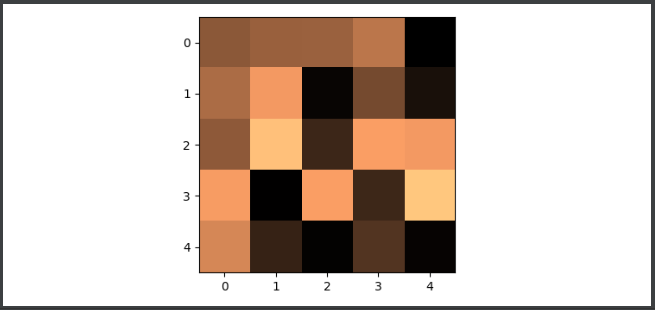# Matplotlib Backend Differences between Agg and Cairo

RendererFile typesDescription
AGGPngRaster graphics − high-quality images using the Anti-Grain Geometry engine
Cairopng, ps, pdf, svgRaster or vector graphics − using the Cairo library

## Steps

• Set the figure size and adjust the padding between and around the subplots.
• Set the backend name as "Agg".
• Create a 5☓5 matrix array using numpy.
• Use imshow() method to display data as an image, i.e., on a 2D regular raster.
• To save the figure, use savefig() method.

## Example

import matplotlib as mpl
import numpy as np
import matplotlib.pyplot as plt
plt.rcParams["figure.figsize"] = [7.50, 3.50]
plt.rcParams["figure.autolayout"] = True
mpl.use("Agg")
data = np.random.rand(5, 5)
plt.imshow(data, interpolation='nearest', cmap="copper")
plt.savefig('agg.png')

## Output